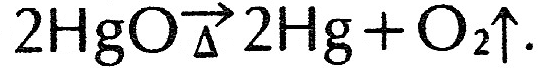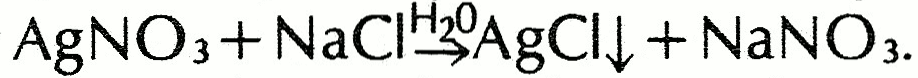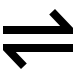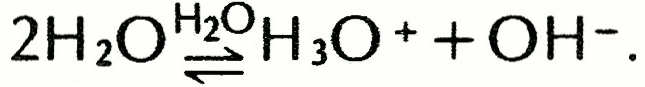Definition: chemical equation from Philip's Encyclopedia

Set of symbols used to represent a chemical reaction. Equations show how atoms are rearranged as a result of a reaction, with reactants on the left-hand side and products on the right-hand side. For example, the formation of magnesium oxide when magnesium burns in oxygen is represented by 2Mg + O2 → 2MgO. The number of atoms of an element on the left-hand side of an equation must equal the number on the right.

Summary Article: chemical equation
From The Columbia Encyclopedia

group of symbols representing a chemical reaction.

Basic Notation Used in Equations

The chemical equation 2H2+O2→2H2O represents the reaction of hydrogen and oxygen to form water. The arrow points in the direction of the reaction—from the reactants (substances that react) toward the product or products. In this case the reactants are hydrogen (written H2 because each molecule consists of two atoms of hydrogen) and oxygen (written O2 because each molecule consists of two atoms of oxygen) and the product is water. The coefficient 2 before the H2 indicates that two molecules of hydrogen take part in the reaction, and the 2 before the H2O indicates that two molecules of water are produced. When no number is written, as in front of the O2, a one is assumed; one molecule of oxygen takes part in the reaction. The equation shows that two molecules of hydrogen react with one molecule of oxygen to form two molecules of water. Because of the relationship between molecules and the mole, the equation also shows that two moles of hydrogen react with one mole of oxygen to form two moles of water. The same sort of relationship holds with the gram-formula weight.

Methodology for Writing an Equation

There are three steps involved in writing a chemical equation. The first step is to decide which substances are the reactants and which are the products. For example, natural gas (cooking gas) burns in air, providing heat and producing no visible products. The natural gas is principally methane, and the portion of the air that reacts (supports combustion) is oxygen. These are the reactants. Products of the reaction are heat and two invisible gases, carbon dioxide and water vapor. We can now write the word equation methane + oxygen → carbon dioxide + water vapor + heat. The next step is to determine the correct formula for each substance and substitute it for the name. The equation now becomes CH4+O2→CO2+H2O. (A notation for heat is often omitted.)

The final step is to balance this equation. As the equation is now written, three oxygen atoms are produced from two, and four hydrogen atoms become only two. This cannot occur, since atoms are not created or destroyed in chemical reactions. The equation is already balanced for carbon, since there is one carbon atom on the reactant side and one carbon atom on the product side. There are four hydrogen atoms in the methane molecule on the reactant side, so there must be four hydrogen atoms in water molecules on the product side (since water is the only product containing hydrogen); thus there must be two water molecules, each containing two hydrogen atoms. The equation can now be written CH4+O2→CO2+2H2O. It is not yet balanced, since there are only two oxygen atoms shown as reactants and four as products. The equation is completely balanced by showing two oxygen molecules (four atoms) as reactants: CH4+2O2→CO2+2H2O.

Additional Symbols Used in Chemical Equations

There are a number of other symbols used in chemical equations. A symbol written above or below the reaction arrow indicates special reaction conditions. For example, when mercuric oxide is heated it decomposes into mercury metal and oxygen gas; this reaction is shown by the equationThe Greek letter delta under the arrow represents the heating. The upward-pointing arrow after the O2 indicates that this product is gaseous and escapes. When a precipitate is formed by a reaction, the substance that precipitates is often followed by a downward-pointing arrow, e.g.,The H2O above the arrow shows that the reaction takes place in the presence of water—in this case, in water solution. The formulas AgNO3, NaCl, and NaNO3 do not represent molecules, since these substances are almost completely ionized in water solution (see ion).

When chemical equilibrium occurs in a reaction, the double arrow () is used instead of the single arrow. For example, liquid water dissociates to form hydronium ions (H3O+) and hydroxide ions (OH). These ions exist in equilibrium with water molecules. The equation isThe sign = is sometimes used in place of the double arrow.

Bibliography
• See , Mathematical Techniques in Chemistry (1975).The Columbia Encyclopedia, © Columbia University Press 2018